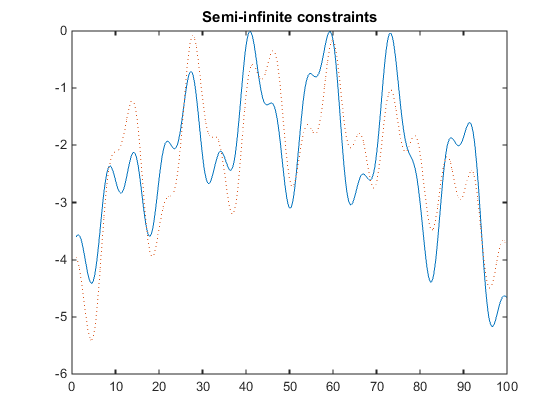## One-Dimensional Semi-Infinite Constraints

Find values of x that minimize

f(x) = (x1 – 0.5)2 + (x2– 0.5)2 + (x3– 0.5)2

where

`$\begin{array}{l}{K}_{1}\left(x,{w}_{1}\right)=\mathrm{sin}\left({w}_{1}{x}_{1}\right)\mathrm{cos}\left({w}_{1}{x}_{2}\right)-\frac{1}{1000}{\left({w}_{1}-50\right)}^{2}-\mathrm{sin}\left({w}_{1}{x}_{3}\right)-{x}_{3}\le 1,\\ {K}_{2}\left(x,{w}_{2}\right)=\mathrm{sin}\left({w}_{2}{x}_{2}\right)\mathrm{cos}\left({w}_{2}{x}_{1}\right)-\frac{1}{1000}{\left({w}_{2}-50\right)}^{2}-\mathrm{sin}\left({w}_{2}{x}_{3}\right)-{x}_{3}\le 1,\end{array}$`

for all values of w1 and w2 over the ranges

1 ≤ w1 ≤ 100,
1 ≤ w2 ≤ 100.

Note that the semi-infinite constraints are one-dimensional, that is, vectors. Because the constraints must be in the form Ki(x,wi) ≤ 0, you need to compute the constraints as

`$\begin{array}{l}{K}_{1}\left(x,{w}_{1}\right)=\mathrm{sin}\left({w}_{1}{x}_{1}\right)\mathrm{cos}\left({w}_{1}{x}_{2}\right)-\frac{1}{1000}{\left({w}_{1}-50\right)}^{2}-\mathrm{sin}\left({w}_{1}{x}_{3}\right)-{x}_{3}-1\le 0,\\ {K}_{2}\left(x,{w}_{2}\right)=\mathrm{sin}\left({w}_{2}{x}_{2}\right)\mathrm{cos}\left({w}_{2}{x}_{1}\right)-\frac{1}{1000}{\left({w}_{2}-50\right)}^{2}-\mathrm{sin}\left({w}_{2}{x}_{3}\right)-{x}_{3}-1\le 0.\end{array}$`

First, write a file that computes the objective function.

```function f = myfun(x,s) % Objective function f = sum((x-0.5).^2);```

Second, write a file `mycon.m` that computes the nonlinear equality and inequality constraints and the semi-infinite constraints.

```function [c,ceq,K1,K2,s] = mycon(X,s) % Initial sampling interval if isnan(s(1,1)), s = [0.2 0; 0.2 0]; end % Sample set w1 = 1:s(1,1):100; w2 = 1:s(2,1):100; % Semi-infinite constraints K1 = sin(w1*X(1)).*cos(w1*X(2)) - 1/1000*(w1-50).^2 -... sin(w1*X(3))-X(3)-1; K2 = sin(w2*X(2)).*cos(w2*X(1)) - 1/1000*(w2-50).^2 -... sin(w2*X(3))-X(3)-1; % No finite nonlinear constraints c = []; ceq=[]; % Plot a graph of semi-infinite constraints plot(w1,K1,'-',w2,K2,':') title('Semi-infinite constraints') drawnow```

Then, invoke an optimization routine.

```x0 = [0.5; 0.2; 0.3]; % Starting guess [x,fval] = fseminf(@myfun,x0,2,@mycon);```

After eight iterations, the solution is

`x`
```x = 0.6675 0.3012 0.4022```

The function value and the maximum values of the semi-infinite constraints at the solution `x` are

`fval`
```fval = 0.0771 ```
```[c,ceq,K1,K2] = mycon(x,NaN); % Initial sampling interval max(K1)```
```ans = -0.0077```
```max(K2) ```
```ans = -0.0812```

A plot of the semi-infinite constraints is produced.This plot shows how peaks in both constraints are on the constraint boundary.

The plot command inside `mycon.m` slows down the computation. Remove this line to improve the speed.